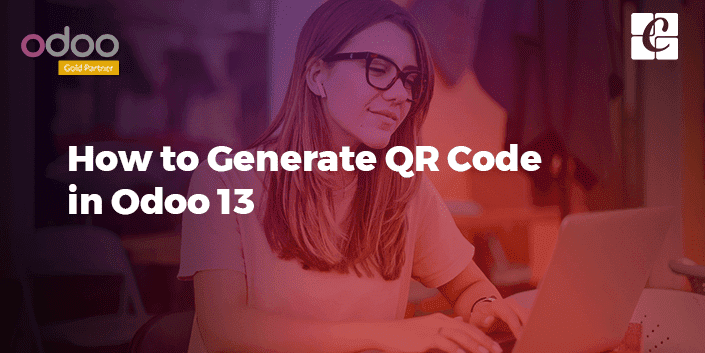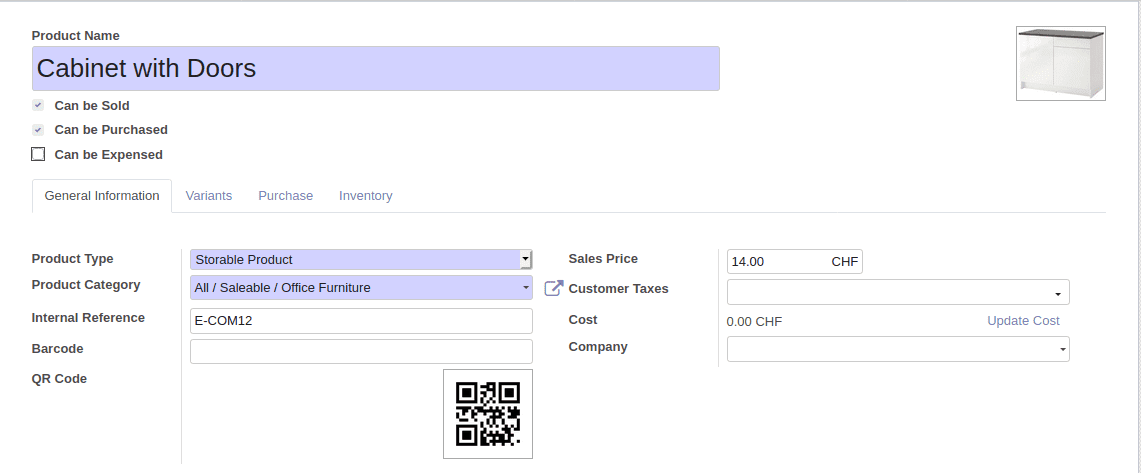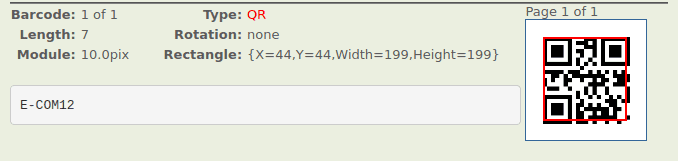By: Akshay Babu

# How to Generate QR Code in Odoo 13

Technical Odoo 13

The first introduction to Quick Response Code or the QR code was in the mid-1990s in Japan. QR code is nothing but a two-dimensional barcode or a matrix barcode. The data can be embedded in the QR code using four standardized encoding modes(numeric, alphanumeric, byte/binary and kanji).

The QR code quickly gained popularity among mass for its faster readability and greater storage capacity. It can be used for product tracking, item identification, time tracking, document managing, and general marketing.

Now let's see how to generate a QR code for a product in odoo. Here we are generating the QR code based on the internal reference of the product. More information on the product can be added to the QR code.

PYTHON CODE:

`import qrcodeimport base64from io import BytesIOfrom odoo import models, fields, apiclass ProductQR(models.Model):    _inherit = "product.template"    qr_code = fields.Binary("QR Code", attachment=True, store=True)    @api.onchange('default_code')    def generate_qr_code(self):        qr = qrcode.QRCode(            version=1,            error_correction=qrcode.constants.ERROR_CORRECT_L,            box_size=10,            border=4,        )        qr.add_data(self.default_code)        qr.make(fit=True)        img = qr.make_image()        temp = BytesIO()        img.save(temp, format="PNG")        qr_image = base64.b64encode(temp.getvalue())        self.qr_code = qr_image`

There is a python library that helps in the generation of QR code image called "QRcode".

Import QR code.

`import qrcode`

Define a binary field to store the QR image.

`class ProductQR(models.Model):    _inherit = "product.template"    qr_code = fields.Binary("QR Code", attachment=True) `

Here the function to generate a QR image is called when there is a change in the internal reference. Any method can be used to call the function to generate the QR code image. Since in this case QR image is generated based on the internal reference value, ‘onchange’ is used.

`    @api.onchange('default_code')    def generate_qr_code(self):        qr = qrcode.QRCode(            version=1,            error_correction=qrcode.constants.ERROR_CORRECT_L,            box_size=10,            border=4,        )        qr.add_data(self.default_code)        qr.make(fit=True)        img = qr.make_image()        temp = BytesIO()        img.save(temp, format="PNG")        qr_image = base64.b64encode(temp.getvalue())        self.qr_code = qr_image`

The QRCode class is used to get more control over the generated QR image.

`     qr = qrcode.QRCode(        version=1,        error_correction=qrcode.constants.ERROR_CORRECT_L,        box_size=10,        border=4,    )`

Version

Version ranges from 1 to 40. Depending on the size of the data to be stored, the version of QR will also change. To determine the QR version automatically, set version to none and while making the code set ‘fit’ parameter as true.

Error Correction

Error correction used for the QR code is controlled by the error correction parameters. There are four constants of error correction in the QRcode package.

1. ERROR_CORRECT_L(7% or less errors can be corrected)

2. ERROR_CORRECT_M(15% or less errors can be corrected)

3. ERROR_CORRECT_Q(25% or less errors can be corrected)

5. ERROR_CORRECT_H(30% or less errors can be corrected)

if not specified ERROR_CORRECT_M would be taken as default

Box Size

The pixel of each box of the QR code is controlled by the Box size parameter

Border

The thickness of the border is controlled by the border parameter. If not set, default value is taken as ‘4’.

The information to be stored in the QR image is added using this method.

qr.make()

This makes the QR code.

Then the image is saved in the png format and later encoded.

`img.save(temp, format="PNG")qr_image = base64.b64encode(temp.getvalue())self.qr_code = qr_image`

XML CODE:

In the XML part, we just have to define the view for that field.

`        <record model="ir.ui.view" id="product_qr_code">           <field name="name">product.template.qr.code</field>           <field name="model">product.template</field>           <field name="type">form</field>           <field name="inherit_id" ref="product.product_template_only_form_view"/>           <field name="arch" type="xml">               <field name="barcode" position="after">                   <field name="qr_code" widget='image' class="oe_avatar" options='{"preview_image":"image_medium"}'/>               </field>           </field>         </record>`

The field defined in the XML should have widget = ‘image’ or else the QR image will not be displayed. Thus we can easily generate a QR code for the product.When the barcode got scanned the following output was obtained.Using this method one can generate a QR code image for any pieces of information in odoo.0

### Recent Posts

#### How to configure SSH

##### Calicut

Cybrosys Technologies Pvt. Ltd.
Neospace, Kinfra Techno Park
Kakkancherry, Calicut
Kerala, India - 673635

##### London

Cybrosys Limited
Alpha House,
100 Borough High Street, London,
SE1 1LB, United Kingdom

##### Kochi

Cybrosys Technologies Pvt. Ltd.
1st Floor, Thapasya Building,
Kochi, India - 682030.

##### Bangalore

Cybrosys Techno Solutions
The Estate, 8th Floor,